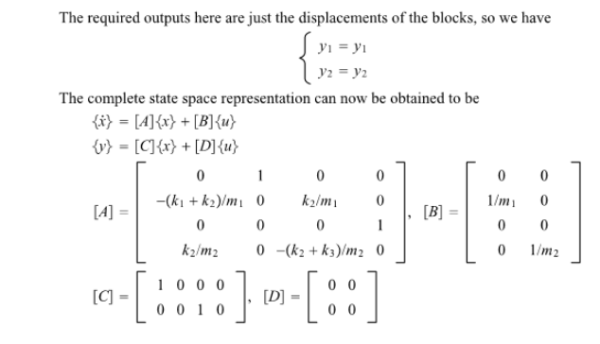Obtain a state-space representation of the mechanical system shown in the figure, where u1 and u2 are the inputs and y1 and y2 are the outputs. The displacements y1 and y2 are measured from their respective equilibrium positions.Obtain a state-space representation of the mechanical system shown in the figure, where u1 and u2 are the inputs and y1 and y2 are the outputs. The displacements y1 and y2 are measured from their respective equilibrium positions.

System Dynamics Page 2 dynamics dynamics dynamics dynamics dynamics dynamics dynamics System dynamics Page 3